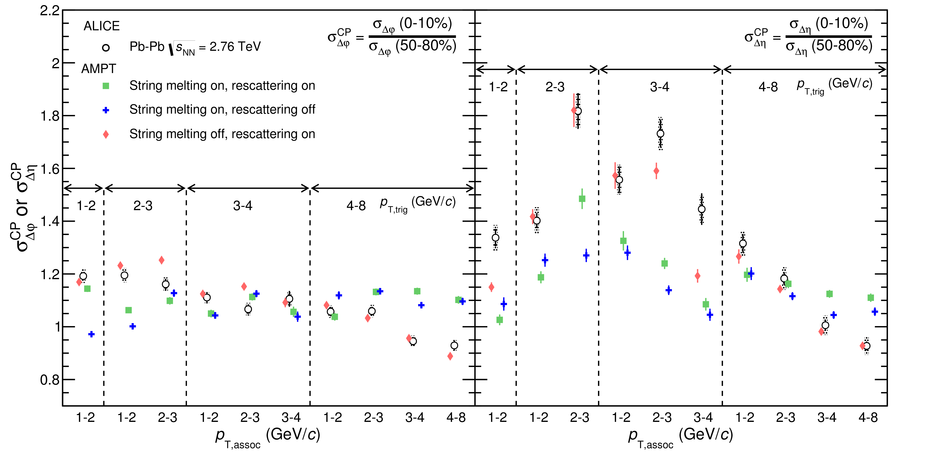# Figure 6

 Ratio of the peak widths in $\Dphi$ (left panel) and $\Deta$ (right panel) observed in central (0--10\%) and peripheral (50--80\%) collisions as a function of $\pt _{\rm ,trig}$ and $\pt _{\rm ,assoc}$ ranges. The data is compared to the different AMPT settings. Note that the $x$-axis combines the $\pta$ and $\ptt$ axis, and therefore, a uniform trend of the values is not expected. Lines indicate statistical uncertainties (mostly smaller than the marker size), while boxes (only for data) denote systematic uncertainties